Technical blog

# Solving the binary paint shop problem with Fire Opal’s QAOA Solver

July 27, 2023
Written by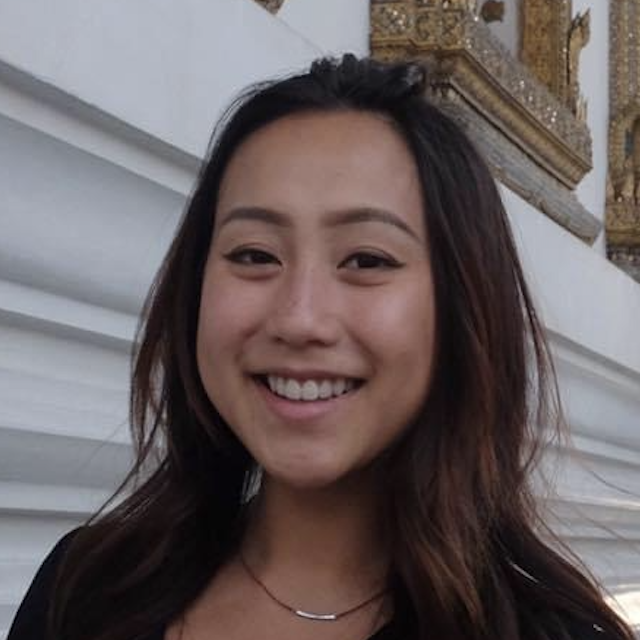Rowen Wu

Fire Opal’s QAOA Solver enables you to get the best performance when running the Quantum Approximate Optimization Algorithm (QAOA) on real quantum hardware. With a single call to solve_qaoa, Fire Opal handles all aspects of the algorithm execution and optimizes for the best performance on real hardware devices.

QAOA is a popular quantum algorithm that can be applied to a range of practical optimization problems, such as portfolio optimization or machine learning. The Binary Paint Shop Problem (BPSP) is one of these important problems, and Fire Opal can easily be applied to determine the correct solution.

## Exploring the binary paint shop problem

BPSP is a standard optimization problem that is described in the context of an automotive paint shop but can also be applied to a variety of industries, including printing and textile manufacturing.

Imagine that your automotive shop has a predefined sequence of cars going down the line to be painted, and each car goes down the line twice. The ordering of the cars cannot be changed due to the timing of other processes and customer orders. You have two colors of paint, red and blue, and you want each car to be painted once with each color.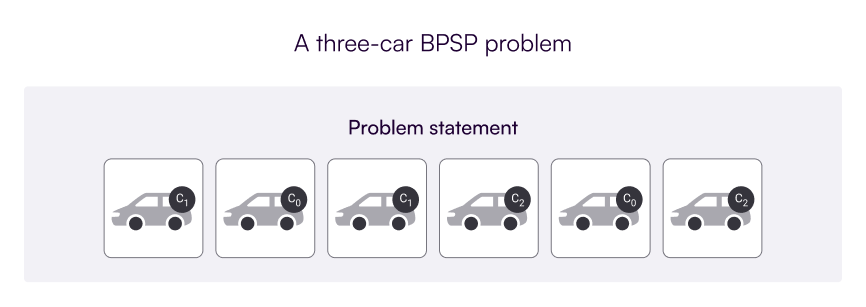Figure 1. A sample BPSP problem for three cars. Cars are labeled C0 through C2, and each car goes down the line exactly twice to be painted.

It’s expensive and time consuming to switch between colors—you have to clean and change all the painting equipment. Therefore, the goal is to determine the optimal way to paint the cars while minimizing the number of color changes.

As part of the problem, you can assume, without loss of generality, that car 0 (C0) must be painted red first. The following image shows the optimal solutions to the previously defined problem, which each require only two paint color changes.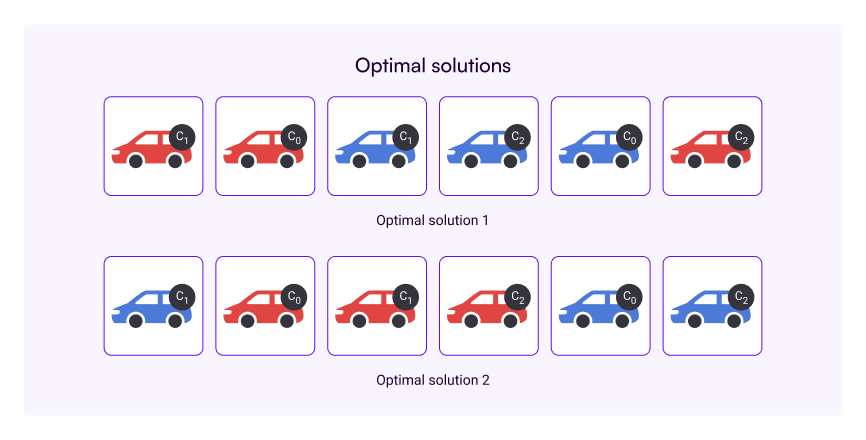Figure 2. The two optimal solutions to the three-car BPSP problem shown in Figure 1. Each solution requires only two color changes to paint all cars in both colors.

This problem is known to be NP-complete and APX-hard. This means that there is no scalable way for classical computers to determine or approximate the right solution.

## Encoding BPSP into QAOA

Research has shown that QAOA can be used to solve BPSP and, with constant depth, is able to beat classical methods, even when the problem scales.

Combinatorial optimization problems, such as BPSP, can be solved using QAOA by first mapping the type of problem to a Hamiltonian which represents the problem as an energy landscape; the Hamiltonian can be used to construct a parameterized quantum circuit. The goal is to find the lowest energy state corresponding to the optimal solution using the outer classical loop. This process iteratively executes the circuit and evaluates the output according to an objective (cost) function. Classical optimization—machine learning—is used to automatically find the best parameters to minimize the cost function.

For BPSP, the cost function is defined by first assigning binary variables to each car, where the value of the variable represents the paint color—for example, ‘0’ for red and ‘1’ for blue. Then a possible solution to the problem is given by a paint bitstring which defines the first paint color for the respective car. In the paint bitstring encoding, the i-th bit is ‘0’ if car i is painted red the first time it appears in the sequence, and blue otherwise.

Then you can add constraints. For each car in the pre-defined sequence, the paint bitstring is used to build up a color sequence, from which you count the number of times the paint color has changed. QAOA uses this “color change count” as the objective to find the optimal paint bitstring which minimizes the number of paint color changes.

Going back to the three car example shown in Figure 1, the paint bitstrings ‘100’ and ‘010’ produce the first and second sequence shown in Figure 2, respectively. The optimal solution bitstring is read from last car to first, such that “Optimal solution 1”— corresponding to the bitstring ‘100’—indicates that car 2 is painted blue first and cars 1 and 0 are painted red first.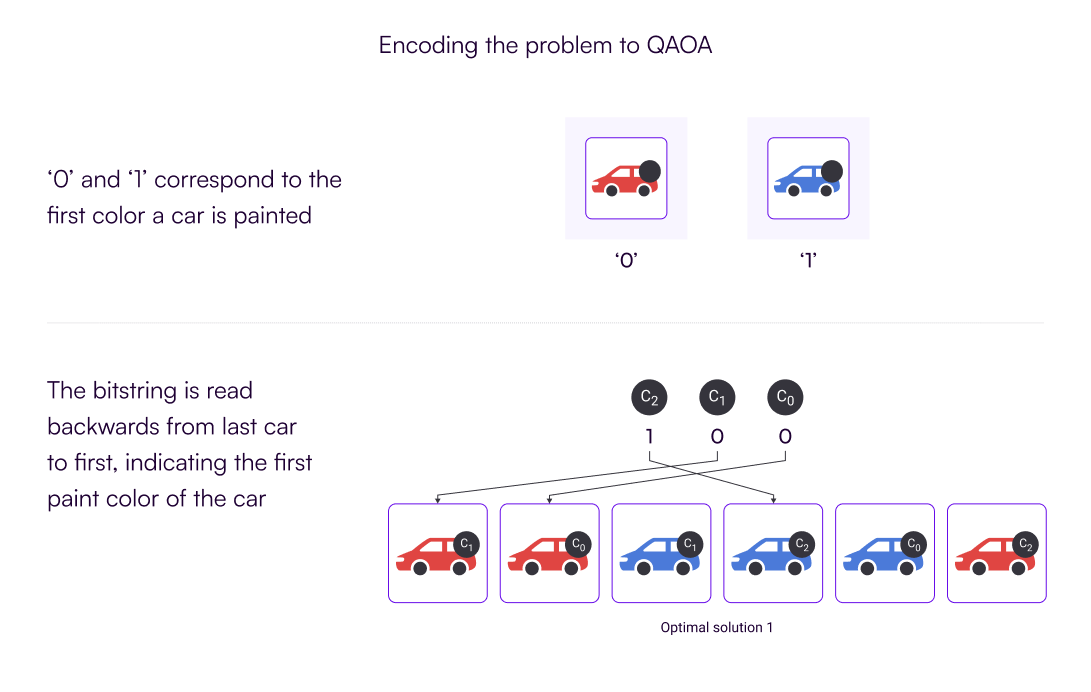Figure 3. The bitstring represents the first color that the car is painted. It reads from last car to first, i.e. C2, C1, C0. The optimal solution bitstring ‘100’ means that C2 is painted blue first; C1 and C0 are painted red first.

The bitstring only needs to represent the first color that each car is painted, since the second color can be inferred. These same bitstrings can also be shortened to ‘10’ and ‘01’ since it’s assumed in the problem statement that car 0 is going to be painted red first, meaning the last bitstring value will always be ‘0’.

## Enabling solutions to complex problems

Unfortunately, despite the promise of quantum computing, when attempting to solve BPSP algorithms using real devices, hardware errors often prevent the algorithm from achieving the correct answer. Oftentimes, even BPSP problems with very few cars result in the wrong solution. That’s why we built Fire Opal’s QAOA Solver.

Fire Opal makes it possible to solve BPSP on a quantum computer and reduces the complexity of running QAOA algorithms by providing a single function that performs the entire execution process.

By leveraging best-in-class error suppression tools and optimizing each part of the implementation to run on hardware, Fire Opal achieves the correct solution with high probability of success!

The following image (Figure 4) shows a randomly generated seven-car BPSP problem. Cars are labeled C0 through C6. The optimal solution is represented by the bitstring ‘000001’, which represents the cars in the order: C6, C5, C4, C3, C2, C1. The first car, C0, is omitted since it’s assumed in the problem definition that car 0 is painted red first.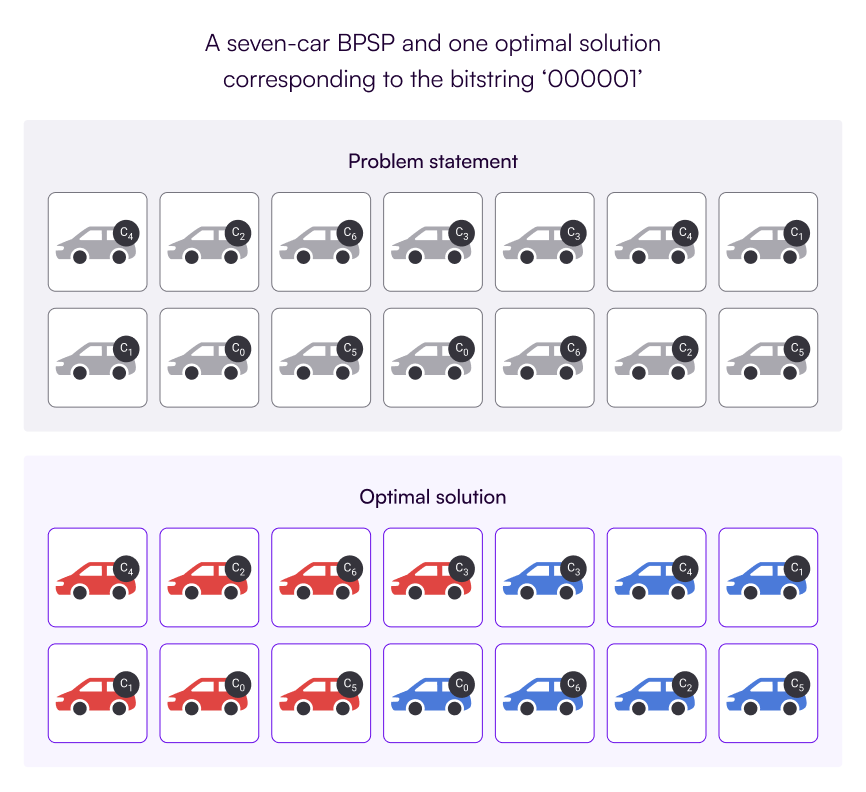Figure 4. Randomly generated problem with 7 cars and resulting optimal solution that corresponds to the bitstring ‘000001’.

When using Fire Opal’s QAOA solver, the results clearly identify the correct solution bitstring “000001” as the solution to the problem, as shown by the highest purple bar in Figure 5.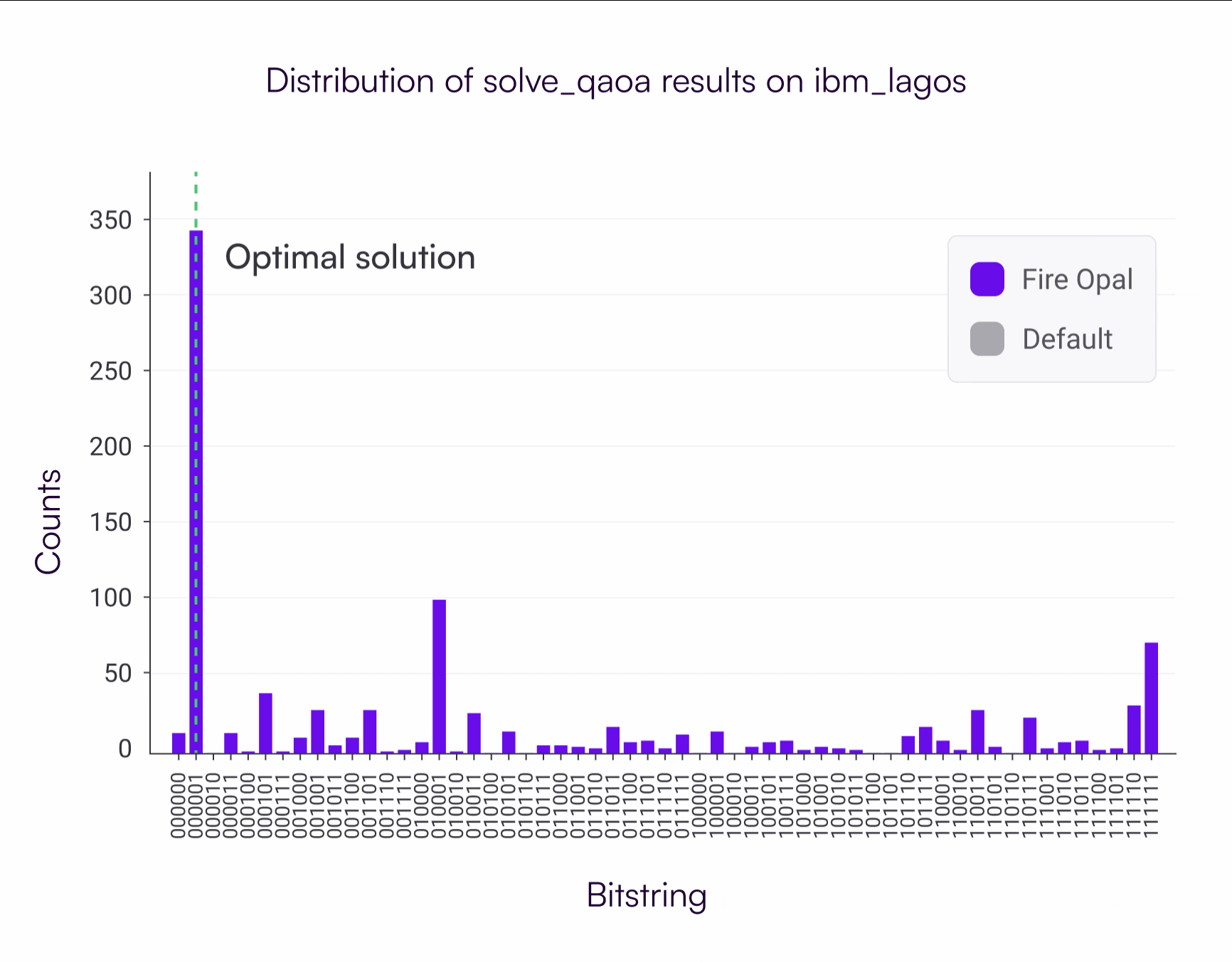Figure 5. Count distribution returned by Fire Opal for a randomly generated seven-car BPSP problem. The highest probability solution generated by Fire Opal was the correct optimal bistring, ‘000001’.

Fire Opal pushes the boundaries of quantum hardware to enable them to solve more complex and interesting problems. A solution for BPSP is just one example that has been unlocked by the hardware-aware capabilities of our QAOA Solver.

You can now obtain good results for all sorts of optimization problems, such as MaxCut, minimum vertex cover, or the vehicle routing problem. Fire Opal also enables costly subroutines like Quantum Phase Estimation to work on real devices without a hitch!

Sign up for Fire Opal to discover how to solve BPSP and experience the benefits firsthand!